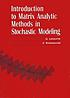# Classify

## Summary

Title:
Introduction to matrix analytic methods in stochastic modeling
Author:
Latouche, G. (Guy); Ramaswami, V.; Society for Industrial and Applied Mathematics
Formats:

Editions:
17
Total Holdings:
287
OCLC Work Id:
25230011
http://classify.oclc.org/classify2/ClassifyDemo?owi=25230011
Most Frequent 519.23 270 Web Dewey
Edition: 22 519.23 12
Most Frequent QA274.7 270 ClassWeb## Editions

Displaying 1 to 17 of 17
Title and AuthorLanguageFormatHoldings TagClass NumberIntroduction to matrix analytic methods in stochastic modelingby Latouche, G. (Guy)eng 149050QA274.7
082519.23

(DDC 21)Introduction to matrix analytic methods in stochastic modelingby Latouche, G. (Guy)eng 67050QA274.7
082519.23

(DDC 21)Introduction to matrix analytic methods in stochastic modelingby Latouche, G. (Guy)eng 17050QA274.7
082519.23Introduction to matrix analytic methods in stochastic modelingby Latouche, G. (Guy)eng 16050QA274.7
082519.23Introduction to matrix analytic methods in stochastic modelingby Latouche, G. (Guy)eng 12050QA274.7
082519.23

(DDC 22)Introduction to matrix analytic methods in stochastic modelingby Latouche, G. (Guy)eng 7050QA274.7
082519.23

(DDC 21)Introduction to matrix analytic methods in stochastic modelingby Latouche, G. (Guy)eng 4082519.233

(DDC 20)Introduction to matrix analytic methods in stochastic modelingby Latouche, G. (Guy)eng 3082519.2

(DDC 0)Introduction to matri× analytic methods in stochastic modelingby Latouche, G. (Guy)eng 2Introduction to matrix analytic methods in stochastic modelingby Latouche, G. (Guy)eng 2082519.23

(DDC 21)

050QA274.7Introduction to matrix analytic methods in stochastic modelingeng 2Introduction to matrix analytic methods in stochastic modelingby Latouche, G. (Guy)eng 1Introduction to matrix analytic methods in stochastic modelingby Latouche, G. (Guy)eng 1082519.2

(DDC 21)Introduction to matrix analytic methods in stochastic modelingby Latouche, G. (Guy)eng 1Introduction to matrix analytic methods in stochastic modelingby Latouche, G. (Guy)eng 1Introduction to matrix analytic methods in stochastic modelingby Latouche, G. (Guy)eng 1Introduction to matrix analytic methods in stochastic modelingby Latouche, G. (Guy)eng 1082519.233

(DDC 20)

Displaying 1 to 17 of 17
.